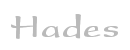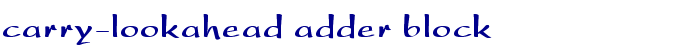TAMS / Java / Hades / applets (print version): contents | previous | nextDescription

This is the 1-bit adder block used in the carry-lookahead adder. It uses two XOR gates to calculate the si sum output bit of the ain, bin and cin (carry in) input bits.

Obviously, this block generates a carry output signal when both the ain and bin inputs are 1. Therefore, a simple AND gate is sufficient to compute the generate-carry signal on the gi output.

Similarly, the OR gate computes the propagate carry signal on the pi output.

Run the applet | Run the editor (via Webstart)

Impressum | 24.11.06